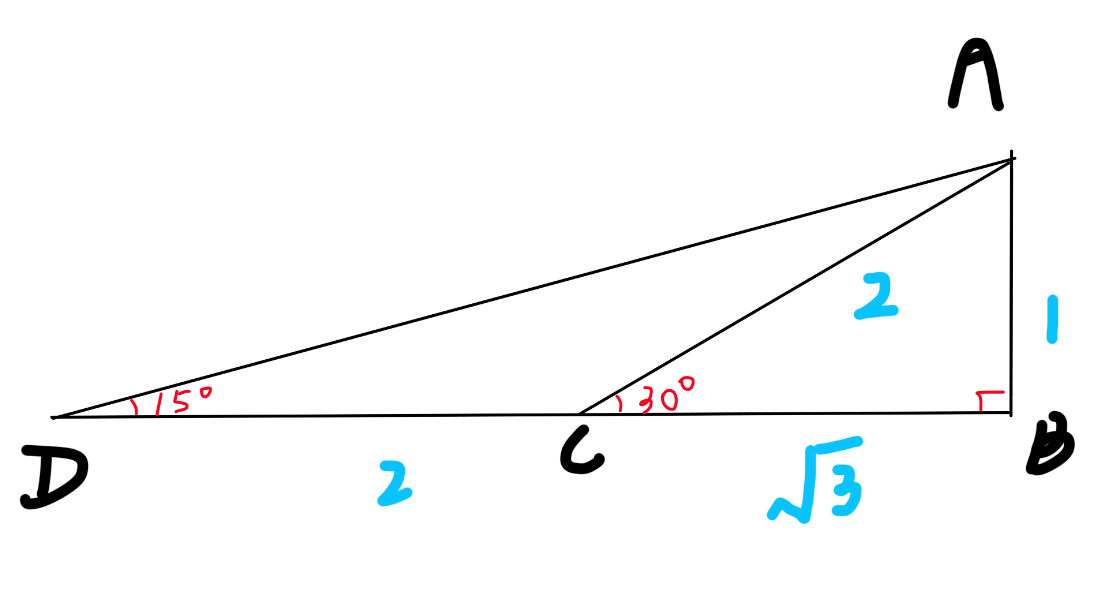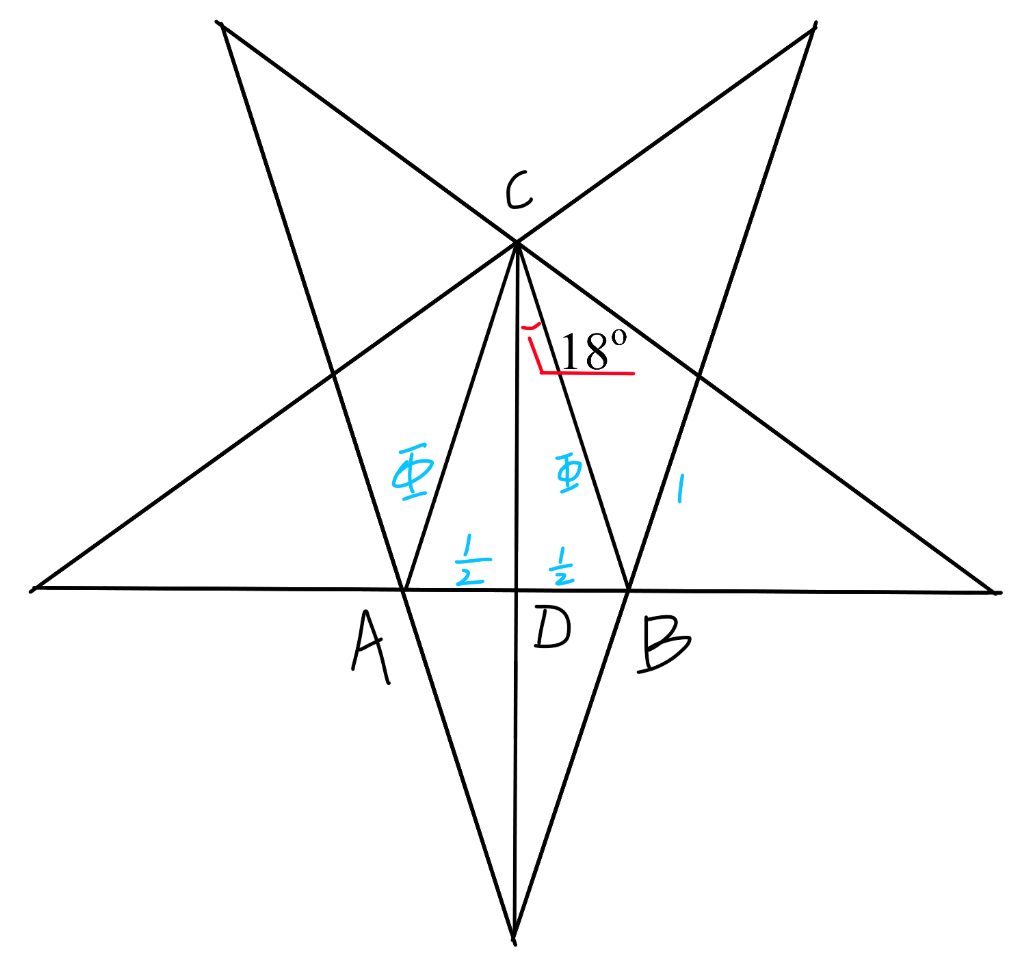# How to find trigonometric values that = radical values?

I have always hoped to rearrange a trigonometric expression into another expression without trigonometric functions. For example, it is common known that $$\sin 60^\circ =\dfrac{\sqrt{3}}{2}$$, but what about other ones?
I have found some already (below) and I wish to find more as this may be helpful when I am to simplify an expression.

## instructions

If you find any typos or improvements comment under my comment that reads: report
The angles I am looking for are integers in range $[0^\circ ,180^\circ]$.
Please do not comment straight away as things will get messy.
If you have found a new solution, comment under my comment below this note in format:
<equation>
<proof———>

## The Transformation table

Resolution found
Formulae used:
$(1)~~~\sin (\pi -A)=\sin A,~~\cos (\pi -A)=-\cos A,~~\tan (\pi -A)=-\tan A.$
$(2)~~~\sin ^2 A+\cos ^2 A=1,~~\tan A=\dfrac{\sin A}{\cos A}.$
$(3)~~~\sin (\dfrac{\pi}{2}+A)=\cos A,~~\cos (\dfrac{\pi}{2}+A)=-\sin A.$
$(4)~~~\sin 2A=2\sin A\cos A.$
$(5)~~~\cos 2A=\cos ^2 A-\sin ^2 A=2\cos ^2 A-1 =1-2\sin ^2 A.$
$(6)~~~\tan 2A=\dfrac{2\tan A}{1-\tan ^2 A}.$
Table 1: integer values in degrees:

 angle $\theta$ in degrees $\sin \theta$ $\cos \theta$ $\tan \theta$ Proof $15^\circ$ $\dfrac{\sqrt{6}-\sqrt{2}}{4}$ $\dfrac{\sqrt{6}+\sqrt{2}}{4}$ $2-\sqrt{3}$ The figure explains everything. In the figure, note that $\triangle ACD$ is isosceles, so $\angle ADB =15^\circ .$$18^\circ$ $\dfrac{\sqrt{5}-1}{4}$ $\dfrac{\sqrt{10+2\sqrt{5}}}{4}$ $\dfrac{\sqrt{25-10\sqrt{5}}}{5}$ We can draw a regular pentagon as follows:Let $AB=1$. From symmetry and some algebra, we get $AC=BC=\dfrac{\sqrt{5}+1}{4}=\phi$ and $AD=BD=1$. Then apply the Pythagorean Theorem on the triangle. Calculations should be tedious but we can arrive at the result. Or we can do this: \begin{aligned} \because \cos 18^\circ \gt 0, \\ \therefore \cos 18^\circ &=\sqrt{1-\sin ^2 18^\circ}\\ &=\sqrt{1-\frac{(5-2\sqrt{5} +1)}{16}}~~~\text{the complete square formula helps} \\ &=\sqrt{\dfrac{10+2\sqrt{5}}{16}} \\ &=\dfrac{\sqrt{10+2\sqrt{5}}}{4}.\end{aligned} It is still a tedious job to calculate $\tan 18^\circ$ though. $20^\circ$ We must use triple angle identities to find the value of $\sin 20^\circ$ and $\cos 20^\circ$. This shall be separated into two sections. $\huge \text{COSINE}$Perhaps we can try $\cos 20^\circ$ first as $\cos (3\times 20^\circ )=\dfrac{1}{2}$. Let $\cos 20^\circ =x$, then $4x^3-3x=\dfrac{1}{2}.$ Therefore $x^3-\frac{3}{4}x-\dfrac{1}{8}=0.$ So we can use the cubic formula to solve this equation. If the function has real coefficients $x^3+ax^2+bx+c=0$, then one real root would be: $\sqrt{-q+\sqrt{r}}+\sqrt{-q-\sqrt{r}}-\frac{a}{3},$ Where $q=\dfrac{1}{27}a^3-\dfrac{1}{6}ab+\dfrac{1}{2}c$, $r=q^2+\dfrac{1}{27}(b-\dfrac{1}{3}a^2)^3$. Plug in $\color{#D61F06} a=0, ~~b=\dfrac{3}{4}, ~~c=\dfrac{1}{8}$ to get the result: $q=\dfrac{1}{16},~~r=\frac{5}{256}.$ $\sqrt{r}=\dfrac{\sqrt5}{16}.$ $\sqrt{-q+\sqrt{r}}+\sqrt{-q-\sqrt{r}}-\frac{a}{3}=\sqrt{\dfrac{\sqrt5-1}{16}}+\sqrt{\dfrac{-\sqrt5-1}{16}}=\dfrac{\sqrt{\sqrt5-1}+\sqrt{-\sqrt5-1}}{2\sqrt{2}}=-0.161092677...$ Check if this is the expected root. $\cos 20^\circ =0.93969262...$, therefore we must try another root but things get complicated. $\huge \text{SINE}$ Let $y=\sin 20^\circ$ and do the same thing. This time $3y-4y^3=\dfrac{\sqrt3}{2}.$ Therefore $y^3-\dfrac{3}{4}y+\dfrac{\sqrt3}{2}=0.$ Again plug in the equation. $30^\circ$ $\dfrac{1}{2}$ $\dfrac{\sqrt{3}}{2}$ $\dfrac{\sqrt{3}}{3}$ N/A $36^\circ$ $\dfrac{\sqrt{10-2\sqrt{5}}}{4}$ $\dfrac{\sqrt{5}+1}{4}$ $\sqrt{5-2\sqrt5}$ Use formulae $(4),(5),(6)$ on the values of $18^\circ$ to get the values. $45^\circ$ $\dfrac{\sqrt{2}}{2}$ $\dfrac{\sqrt{2}}{2}$ $1$ N/A $54^\circ$ $\dfrac{\sqrt5+1}{4}$ $60^\circ$ $\dfrac{\sqrt{3}}{2}$ $\dfrac{1}{2}$ $\sqrt{3}$ N/A $72^\circ$ $\dfrac{\sqrt{5}-1}{4}$ $75^\circ$ $\dfrac{\sqrt{6}+\sqrt{2}}{4}$ $\dfrac{\sqrt{6}-\sqrt{2}}{4}$ $2+\sqrt{3}$ Apply formula $(3)$ and let $A=15^\circ$ to get the sine and cosine. Then we can find tangent easily. $90^\circ$ $1$ $0$ undefined N/A $105^\circ$ $\dfrac{\sqrt6 +\sqrt2}{4}$ $\dfrac{\sqrt2 -\sqrt6}{4}$ $-2-\sqrt3$ Use formula $(1)$ on the values of $75^\circ$ to get the values. $108^\circ$ $\dfrac{1-\sqrt{5}}{4}$ $120^\circ$ $\dfrac{\sqrt3}{2}$ $-\dfrac{1}{2}$ $-\sqrt3$ Use formula $(1)$ on the values of $60^\circ$ to get the values. $126^\circ$ $135^\circ$ $\dfrac{\sqrt{2}}{2}$ $-\dfrac{\sqrt{2}}{2}$ $-1$ Use formula $(1)$ on the values of $45^\circ$ to get the values. $144^\circ$ $\dfrac{\sqrt{10-2\sqrt{5}}}{4}$ $\dfrac{-\sqrt{5}-1}{4}$ $-\sqrt{5-2\sqrt5}$ Use formula $(1)$ on the values of $36^\circ$ to get the values. $150^\circ$ $\dfrac{1}{2}$ $-\dfrac{\sqrt3}{2}$ $-\dfrac{\sqrt3}{3}$ Use formula $(1)$ on the values of $30^\circ$ to get the values. $162^\circ$ $\dfrac{\sqrt5-1}{4}$ $-\dfrac{\sqrt{10+2\sqrt{5}}}{4}$ $-\dfrac{\sqrt{25-10\sqrt{5}}}{5}$ Use formula $(1)$ on the values of $18^\circ$ to get the values. $165^\circ$ $\dfrac{\sqrt6-\sqrt2}{4}$ $\dfrac{-\sqrt6-\sqrt2}{4}$ $\sqrt3-2$ Use formula $(1)$ on the values of $15^\circ$ to get the values.

Table 2: fraction values in radians:Note by Jeff Giff
5 months, 2 weeks ago

This discussion board is a place to discuss our Daily Challenges and the math and science related to those challenges. Explanations are more than just a solution — they should explain the steps and thinking strategies that you used to obtain the solution. Comments should further the discussion of math and science.

When posting on Brilliant:

• Use the emojis to react to an explanation, whether you're congratulating a job well done , or just really confused .
• Ask specific questions about the challenge or the steps in somebody's explanation. Well-posed questions can add a lot to the discussion, but posting "I don't understand!" doesn't help anyone.
• Try to contribute something new to the discussion, whether it is an extension, generalization or other idea related to the challenge.

MarkdownAppears as
*italics* or _italics_ italics
**bold** or __bold__ bold
- bulleted- list
• bulleted
• list
1. numbered2. list
1. numbered
2. list
Note: you must add a full line of space before and after lists for them to show up correctly
paragraph 1paragraph 2

paragraph 1

paragraph 2

[example link](https://brilliant.org)example link
> This is a quote
This is a quote
    # I indented these lines
# 4 spaces, and now they show
# up as a code block.

print "hello world"
# I indented these lines
# 4 spaces, and now they show
# up as a code block.

print "hello world"
MathAppears as
Remember to wrap math in $$ ... $$ or $ ... $ to ensure proper formatting.
2 \times 3 $2 \times 3$
2^{34} $2^{34}$
a_{i-1} $a_{i-1}$
\frac{2}{3} $\frac{2}{3}$
\sqrt{2} $\sqrt{2}$
\sum_{i=1}^3 $\sum_{i=1}^3$
\sin \theta $\sin \theta$
\boxed{123} $\boxed{123}$

Sort by:

## Reports

Post problems or improvements here

- 5 months, 2 weeks ago

## Solutions

Post solutions here

- 5 months, 2 weeks ago

If any picture isn’t functioning, tell me below this comment :)

- 5 months, 2 weeks ago

I don’t see any picture in this note at all?!! , I don’t even see any space for any picture, is there a picture supposed to be there for the proof

- 5 months, 2 weeks ago

Well in the buttons there are pics I think?

- 5 months, 2 weeks ago

Previously I saw no pic in the first button, but now I can, but I can’t see any pic in the second button

- 5 months, 2 weeks ago

And I really like that proof, I used to always run around quadratic formulae if I forgot the value of sin\cos 15 and it’s multiples

- 5 months, 2 weeks ago

You’ll also like the proof for $\sin 18^\circ$ :)

- 5 months, 2 weeks ago

It’s very nice to see it has a clear geometry approach, takes a cosine law to find $\phi$ and the rest is easy, but it does have the same complexity as $\sin 36\degree = \cos 54\degree$ approach

- 5 months, 2 weeks ago

I also knew this one before: $\sin(1.5 \degree)=\dfrac{\sqrt{2\sqrt{2}-\sqrt{2\sqrt{2}+\sqrt{3+\frac{1+\sqrt{5}}{2}+\sqrt{3(\frac{1+\sqrt{5}}{2})\sqrt{5}}}}}}{2\sqrt{2}}..........$

Proof: (Skipping some of the calculations) $\sin 5\theta=(5+16\sin^4\theta-20\sin^2\theta)\sin\theta$ let $\theta=36\degree$ $(5+16\sin^436\degree-20\sin^236\degree)\sin 36\degree=\sin 180\degree=0$ $\because \sin 36\degree\cancel{=}0$ $\therefore 5+16\sin^436\degree-20\sin^236\degree=0$ let $x=\sin^236\degree<0.5$ $\Rightarrow 16x^2-20x+5=0$ $\Rightarrow x=\frac{5^+_-\sqrt{5}}{8}$ $\because \frac{5+\sqrt{5}}{8}>0.5$ $\therefore x=\frac{5-\sqrt{5}}{8}$ $\Rightarrow \sin 36\degree=\frac{\sqrt{5-\sqrt{5}}}{2\sqrt{2}}$ $\Rightarrow \cos 36\degree=\frac{\sqrt{3+\sqrt{5}}}{2\sqrt{2}}$ $\Rightarrow \sin 6\degree=\sin(36\degree-30\degree)$ $=\sin 36\degree\cos 36\degree-\sin 30\degree\cos 36\degree$ $=\dfrac{\sqrt{15-3\sqrt{5}}-\sqrt{3+\sqrt{5}}}{4\sqrt{2}}$ Now using the identity : $\sin\frac{\theta}{2}=^+_-\dfrac{\sqrt{1-\sqrt{1-\sin^2\theta}}}{\sqrt{2}}$ and $0<\sin 1.5\degree<\sin 3\degree$ we can find $$

- 5 months, 2 weeks ago

You can use the angle addition equations to obtain more that are multiples of $15°$ and $18°$:

$\sin 45° = \cfrac{\sqrt{2}}{2}$, $\cos 45° = \cfrac{\sqrt{2}}{2}$, $\tan 45° = 1$

$\sin 60° = \cfrac{\sqrt{3}}{2}$, $\cos 60° = \cfrac{1}{2}$, $\tan 60° = \sqrt{3}$

$\sin 75° = \cfrac{\sqrt{2} + \sqrt{6}}{4}$, $\cos 75° = \cfrac{\sqrt{6} - \sqrt{2}}{4}$, $\tan 75° = 2 + \sqrt{3}$

$\sin 90° = 1$, $\cos 90° = 0$

$\sin 105° = \cfrac{\sqrt{2} + \sqrt{6}}{2}$, $\cos 105° = \cfrac{\sqrt{2} - \sqrt{6}}{2}$, $\tan 105° = -2 - \sqrt{3}$

$\sin 120° = \cfrac{\sqrt{3}}{2}$, $\cos 120° = -\cfrac{1}{2}$, $\tan 120° = -\sqrt{3}$

$\sin 135° = \cfrac{\sqrt{2}}{2}$, $\cos 135° = -\cfrac{\sqrt{2}}{2}$, $\tan 135° = -1$

$\sin 150° = \cfrac{1}{2}$, $\cos 150° = -\cfrac{\sqrt{3}}{2}$, $\tan 150° = -\cfrac{\sqrt{3}}{3}$

$\sin 165° = \cfrac{\sqrt{6} - \sqrt{2}}{2}$, $\cos 165° = \cfrac{-\sqrt{2} - \sqrt{6}}{4}$, $\tan 165° = \sqrt{3} - 2$

$\cos 36° = \cfrac{1 + \sqrt{5}}{4}$

$\sin 54° = \cfrac{1 + \sqrt{5}}{4}$

$\cos 72° = \cfrac{\sqrt{5} - 1}{4}$

$\cos 108° = \cfrac{1 - \sqrt{5}}{4}$

$\sin 126° = \cfrac{1 + \sqrt{5}}{4}$

$\cos 144° = \cfrac{-1 - \sqrt{5}}{4}$

$\sin 162° = \cfrac{\sqrt{5} - 1}{4}$

If you allow for radicals inside radicals, there are several more that can be obtained using half angle formulas (for example, $\sin 22.5° = \cfrac{\sqrt{2 - \sqrt{2}}}{2}$)

Other angles are roots of polynomials, for example, $\cos\bigg(\cfrac{180°}{7}\bigg)$ is a root of $8x^3 - 4x^2 - 4x + 1$ (see here), but this doesn't come out to a clean rational number in radical form.

- 5 months, 2 weeks ago

Could you give a hint on how to prove the $\cos{\frac π 7}$ part, should I be using the $\cos 3 θ$ formula or should I be using $(e^{\frac {iπ}7})^7$

- 5 months, 2 weeks ago

Check out this website here.

Looks like either approach could work.

- 5 months, 2 weeks ago

Thank you, never expected both would work

- 5 months, 2 weeks ago

Well luckily I have a book including one tedious formula for cubic functions :D only it gives nested radicals :(

- 5 months, 2 weeks ago

Are you talking about The Cubic Formula?

- 5 months, 2 weeks ago

Yes :)

- 5 months, 2 weeks ago

Best is the Newton–Raphson method it is applicable for all polynomial equations of any finite degree (mostly gives approximated value not exact)

- 5 months, 2 weeks ago

Ah yes, but again it only works for approximations :) to require precise results things get tedious :) I suppose I like Cardano’s method better :)

- 5 months, 2 weeks ago

- 5 months, 2 weeks ago

Check this

- 5 months, 2 weeks ago

Thanks!

- 5 months, 2 weeks ago